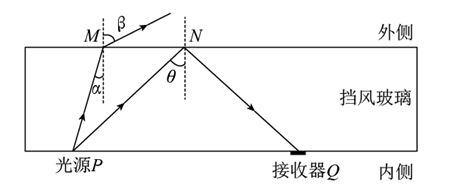【解析】根据光的折射定律有
$$n=\frac{\sin \beta}{\sin \alpha}$$

$$\sin \theta=\frac{1}{n}$$

$$\sin \theta=\frac{\sin \alpha}{\sin \beta}$$
①点击 收藏 此题， 扫码注册关注公众号接收信息推送（一月四份试卷,中1+高2+研1）
② 程序开发、服务器资源都需要大量的钱，如果你感觉本站好或者受到到帮助，欢迎赞助本站,赞助方式：微信/支付宝转账到 18155261033

 ①此题难易度如何 ②此题推荐度如何 确定